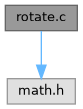GRASS GIS 8 Programmer's Manual  8.2.2dev(2023)-3d37ee165c
rotate.c File Reference

GIS Library - rotate. More...

`#include <math.h>`
Include dependency graph for rotate.c:Go to the source code of this file.

## Macros

#define RpD   ((2 * M_PI) / 360.) /* radians/degree */

#define D2R(d)   (double)(d * RpD) /* degrees->radians */

#define R2D(d)   (double)(d / RpD) /* radians->degrees */

## Functions

void G_rotate_around_point (double X0, double Y0, double *X1, double *Y1, double angle)
Rotate point (double version) More...

void G_rotate_around_point_int (int X0, int Y0, int *X1, int *Y1, double angle)
Rotate point (int version) More...

## Detailed Description

GIS Library - rotate.

(C) 2001-2014 by the GRASS Development Team

This program is free software under the GNU General Public License (>=v2). Read the file COPYING that comes with GRASS for details.

Definition in file rotate.c.

## ◆ D2R

 #define D2R ( d ) (double)(d * RpD) /* degrees->radians */

Definition at line 18 of file rotate.c.

## ◆ R2D

 #define R2D ( d ) (double)(d / RpD) /* radians->degrees */

Definition at line 19 of file rotate.c.

## ◆ RpD

 #define RpD   ((2 * M_PI) / 360.) /* radians/degree */

Definition at line 17 of file rotate.c.

## ◆ G_rotate_around_point()

 void G_rotate_around_point ( double X0, double Y0, double * X1, double * Y1, double angle )

Rotate point (double version)

Given a point, angle, and origin, rotate the point around the origin by the given angle. Coordinates and results are double prec floating point.

Parameters
 X0 X component of origin (center of circle) Y0 Y component of origin (center of circle) [out] X1 X component of point to be rotated (variable is modified!) [out] Y1 Y component of point to be rotated (variable is modified!) angle in degrees, measured CCW from east

Definition at line 35 of file rotate.c.

Referenced by G_rotate_around_point_int().

## ◆ G_rotate_around_point_int()

 void G_rotate_around_point_int ( int X0, int Y0, int * X1, int * Y1, double angle )

Rotate point (int version)

Given a point, angle, and origin, rotate the point around the origin by the given angle. Coordinates are given in integer and results are rounded back to integer.

Parameters
 X0 X component of origin (center of circle) Y0 Y component of origin (center of circle) [out] X1 X component of point to be rotated (variable is modified!) [out] Y1 Y component of point to be rotated (variable is modified!) angle in degrees, measured CCW from east

Definition at line 62 of file rotate.c.

References G_rotate_around_point(), and x.